Pathways To Learning... Since 2005 Hong Kong Registered School 566985

Menu

# E - e to extremum - Mathematics Dictionary

A   B   C   D   E   F   G   H   I   J   K   L   M   N   O   P   Q   R   S   T   U   V   W   X   Y   Z

e: 1. A transcendental constant with a value of 2.71828182846... represented by the letter e, for exponential functions. It is base of the natural logarithm and the base of THE exponential function (whose derivative is itself).

eccentric: The deviation from being perfectly circular.

eccentric angle: For a point on an ellipse, the line parallel to the minor axis intersects the auxilliary circle at 2 points. The radius of the circle that the closer of these 2 points make with the major axis is the eccentric angle..

eccentricity: A parameter for a conic section - the constant ratio of the distance from the focus to the distance from the directrix for any points on the conic section. Usually denoted by e.

echelon form: A matrix derived from applying row (or column) operations such that the number of leading zero entries in each rwo (or column) must be either greater than the row (or column) before, or the same number as before in the case where both the row (or column) itself and the one before contain all zero entries..

edge: A line segment between two vertices in a graph (graph theory) or geometric shape..

effect variable: Another class="d-title" name for a dependent variable.

efficiency: A measure in statistics of the sample size needed to achieve a specified level of accuracy.

eigenvalue: The ratio of the magnitudes of an eigenvector after the transformation to before.

eigenvector: A vector whose direction remains unchanged after a linear transformation.

elastic collision: A collision of 2 bodies where kinetic energy is not lost.

elastic constants: Parameters for the properties or behaviour of components of a system relative to other components (due to its material, construction etc.) under influence of forces.

elasticity: Property of a system whose configuration is dependent on the presence of an external force (or influence/factor).

electromagnetic wave: Electromagnetism as it is considered to exhibits wave-like behaviour as it travels.

element: 1. A member of a set.

2. Components of a matrix.

elementary matrix: Invertible matrices which represent elementary row (or column) operations when left-multiplied (right-multiplied).

elementary matrix operation: One 2 sets (row or column) of three types of operations on matrices which corresponds to left-multiplying (right-multiplying) the matrices, which leave the solution set unchanged.

1. Multiplying a row (column) by a scalar factor

2. Adding a scalar multiple of a row (column) to another row (column)

3. Swapping all entries in 2 rows (columns) with each other

elimination: The removal of variables from a system fo equations through substitution or other operations on the equations.

ellipse: A conic section with eccentricity strictly between 0 and 1. Equivalently, an stretched (or squashed) circle. Also equivalent to the cross section of a cone with a plane where the angle between the plane and the base of the cone is less than the angle between the slanted height and the base, provided that the plane does not intersect with the base, as shown below.ellipsoid: A stretched or squashed sphere.

ellipsoidal: With the properties of or related to an ellipsoid.

elliptical: With the properties of or related to an ellipse.

elliptic curve: A smooth curve of the form,where the discriminant is not zero.

elongation: Transformation of a geometric figure such that distances increase along one reference axis.

empirical: Of or related to information obtained by observations.

empirical distribution function: A distribution function as suggested by the sample.

empty set: The set with no elements. (Any two sets with no elements must be the same set due to the Axiom of Extensionality.)

end behaviour: The properties of a graph on the real number line, or of a function of one real variable, as the real number tends towards either/both positive and negative infinity.

end point: 1. One of 2 numbers which defines a closed interval.

2. One of 2 vertices which defines an edge.

enlargement: A linear transformation relative to a point (called the centre) with a scale factor, where all points will be tranformed to another point in the same diection, relative to the centre, where the ratio of the distance from the centre after transformation to the distance before transformation is the scale factor. A negative scale factor would indicate that the point after transformation is on the opposite side of the centre to the point before transformation.

enneagon: A nine-sided polygon also known as a nonagon. The prefix ennea- is Greek, just as the case fr pentagona nd hexagon, thus arguably more consistent even though it is nonetheless less common.

entailment: A relation between sentences in meta-logic.

entire function: A complex valued function that is holomorphic over all complex numbers.

enumerable: Also known as denumerable and countable.

envelope: A curve which is tangent to (parallel at the point of intersection) all members of a set (usually family) of curves..

epicycle: The outer circle in producing the locus in an epicycloid.

epicyclic: Of or related to an epicycloid.

epicycloid: The locus of the point fixed on the circumference of a circle which rolls around (wthout slipping) the outside of another, fixed circle.

epitrochoid: The locus of the point fixed anywhere on the a circle which rolls around (wthout slipping) the outside of another, fixed circle.

equals sign: The symbol = placed between two mathematical sentences to assert their sameness.

equate: To assert the sameness of two quantities.

equate coefficients: Process of finding coefficients of a polynomial through the fact that the coefficients of the terms of a polynomial represented in any way must be the same.

equation: A mathematical sentence asserting the sameness of two quantities through the use of an equals sign.

equation of motion: Any equations related to the motion of objects.

For example the differential equation,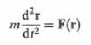known as Newton's second law (of motion).

Or the equations of motion under constant acceleration,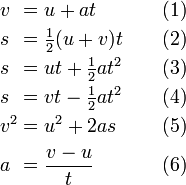regarding the quantities s displacement, u initial velocity, v final velocity, a (constant) acceleration and t time. In this case, any of the equations can be derived from any combination of 2 others. (While (1) and (6) are different representation of the same equation.)

equiangular: Having equal angles. (Describing a geometric figure, commonly a polygon.)

equiangular spiral: A spiral where the tangent always maintain the same angle from the line between the point and a reference point. More commonly known as a logarithmic spiral. It is the path of a moth flying, what seems to be, towards a light source.

equidistant: Of equal distances to some reference geometric figure.

equilateral: Having edges of equal lengths. (Describing a geometric figures, commonly a polygon.).

equilateral polygon: A polygon which has sides of equal length, but not necessarily all the same angles. (If an equilateral polygon is also equiangular it is called a regular polygon.)

equilibrant: A label, rather than a technical definition, of a force which sustains the equilibrium of a system..

equilibrium: The state of a system where the influences collectively negate the effects of each other.

equinumerable: The relation between 2 sets which are/can be bijective.Also known as equipotent - "equal in effect" or equipollent "equal in power".

equivalence: Two objects having the same properties (such as truth value) regardless of the properties of other objects.

equivalence principle: Several related concepts that equates systems of different frames of reference.

equivalence, properties of: A binary relation R is an equivalence relation if the relation is:

Reflexive so that all objects are related to itself: x R x for all x

Symmetric so that if x R y implies that y R x.

Transitive so that if x R y and y R z then x R z.

equivalent: The property of two objects with equivalence.

equivalent systems of equations: Systems of equations with the same set of solutions.

eradius: The radius of a circle (known as an excircle) outside a triangle where the (infinite) lines coinciding with the three sides are tangents of the circle. Also known as an exradius.

erf: A shorthand for the error function.

error: The difference or maximum possible difference between an observation/estimation/representation and the actual value.

error function: A function used for calculating the probability of an observation lying within a certain interval for a normally distributed random variable, defined as the integralerror mean square: A way of measuring the difference between a value implied by an estimator and the actual value.

errors of the first and second kind: Also known as Type I and Type II error in hypothesis testing. thesis testing.

escape speed: More commonly known as escape velocity even though it is a scalar quantity. It is the speed an object needs to travel in, given its distance from the object (or the centre of gravity of the object) so that the kinetic energy is greater than the difference in gravitational potential energy between that point and the gravitational potential energy of a point at infinity.

essential discontinuity: A discontinuity where the left-hand limit or the right-hand limit doesn't exist (or neither exists). Some authors consider a jump discontinuity to be an essential discontinuity, which makes the definition of essential discontinuity any discontinuity that is not a removable discontinuity.

estimation: Calculation of a parameter via estimators, based on observations.

estimator: A statistic which is intended to estimate a quantity.

Euclidean algorithm: An algorithm for finding the HCF of two positive integers.

Euclidean construction: Geometric construction using only straight edges and compasses. Also known as geometric construction or simply a straightedge and compass construction..

Euclidean geometry: The geometry system defined by the following axioms and "notions".

The 5 common notions were:

(1) Things which are equals of the same (third) thing are equal to each other.

(2) If equals are to be added to equals, the sums are also equal equal.

(3) If equals are to be subtracted from equals, the remainders are also equal.

(4) Things which coincide are equal to each other.

(5) The whole is greater than the parts.

Euclid's postulates were:

(1) It is always possible to construct a straight line between any two distinct points.

(2) Any line segments can be extended indefinitely in both directions (to form a line)

(3) It is always possible to construct a circle given any point (as the centre) and any distance (as the radius)

(4) All right angles are the same as each other.

(5) For a straight line L0 which intersects two other straight lines, L1 and L2, there are two pairs of angles formed between L0 and L1 as well as L0 and L2 on the same side of L0. If L1 and L2 were to be extended indefinitely on the side of L0 where the sum of the two angles add to less than half a revolution (180o) then those extensions intersect each other - This is also known as the parallel postulate, one important distinction between Euclidean geometry and non-Euclidean geometry.

Euclidean norm: The usual norm for finding the magnitude of a vector in Rn - the square root of the sum of the orthogonal components of a vector.

Euclid's Theorem: A theorem which states that there are infinitely many primes.

The proof works by reductio ad absurdum (an indirect proof) which states that, if there are indeed finitely many prime numbers, then the number which is one greater than the sum of those (all of the) finitely many prime numbers cannot be completely divided by any of the prime numbers - which makes it a prime number by definition. Thus, no finite list of prime numbers can ever be complete, and thereby shows that the assumption of a finite list of prime numbers is absurd. Hence there must be infinitely many prime numbers.

euler line: A line which passes through the orthocentre, centroid, and circumcentre and orthocentre of a non-equilateraltriangle.

Euler's constant: Usually represented by the symbol γ, also known as the Euler-Mascheroni constant. It can be calculated by.Euler's formula: An identity relating the constant e to the trigonometric functions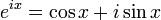Euler's identity: Also known as Euler's Equation. An identities relating five constant, noted for its mathematical elegance, derived from Euler's Formula.Euler's theorem (for polyhedra): A result which states the the number of faces added to the number of vertices is 2 more than the number of edges for all convex polyhedra.

even function: A function whose value is the same by substituting the argument with its additive inverse. i.e. f(x) = f(-x) for all x values.

The graph f such a function is necessarily reflective symmetric about the line x = 0 (i.e. the y-axis).

even number: An integer congruent to 0 modulo 2 - i.e. an integer which can be divided by 2 with an integer quotient and no remainder.

event: Certain subsets of the set of all outcomes (i.e. the sample space). In the case the sample space is finite, there are no restrictions as to which subset are events.

evolution: Also known as extraction, the process of finding a root of a number. The opposite process is called involution.

exa-: SI preix meaning one quadrillion (British long scale) or one quintillion (American short scale) - 1 000 000 000 000 000 000.

exact division: Division where the quotient is "whole" (an integer or a polynomial) with no remainders.

excentre: The centre of an excircle. Also known as an ecentre.

excircle (escribed circle): A circle lying outside a given triangle where the (inifinite) lines coinciding with the 3 sides are tangents to the circle. Also known as an ecircle.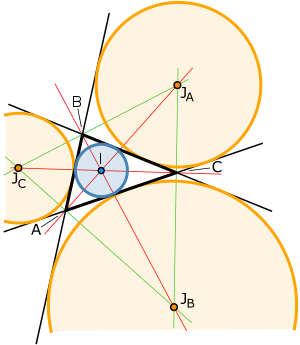The orange circles above are the excircles.

existential quantifier: Represented by the symbol ∃, and read as "there is/exists", it qualifies the the mathematical sentence by asserting that the statement is not false for all elements of the domain. (i.e. that it is true for at least some elements.)

exp: A shorthand for a (the) exponential function. Considered as a unary operator, it raises the constant e to the power of the argument; while considered as a binary operator, it raises the first number to the power of the second number.

expand: To go through the process of expansion.

expanded number: Writing a number as the sum of multiples of powers of the base.

expansion: Rewriting a mathematical expression in an equivalent form that is considered to be longer or more complicated

expectation: Also known as an expected value. The average (mean) of the values of a random variable weighted by the probability (or probability density of them occurring.

It is caluclaued by the summationfor discrete variables and the integralfor continuous variables.

It is represented by E(X) for the random variable X and it can be thought of as the long term average value that appears.

experiment: A controlled process for making observations and gathering data.

explanatory variable: Another class="d-title" name for an independent variable.

explement: Also known as a conjugate angles, it is the angle with which completes a revolution.

explicit differentiation: The process of differentiating an explicit function.

explicit function: A function specified in such a way that the value (output) can be directly calculated from other variables (arguments or inputs).

exponent: The superscript (index) on a mathematical expression that indicates the (extension of the) idea of repeated multiplications. Also known as an index or power.

exponential curve: A curve or a translation of the base curve with equation y = ax.

exponential decay: A exponential model for decay of a quantity for which the rate of decay is directly proportional to the amount present. This model is used for phenomena such as radioactivity or depreciation.

exponential distribution: A probability distribution of the amount of time between 2 consecutive independent singly events where the events are known (or at least assumed) to occur continuously with a constant rate.

The probability density function for a continuous random variable distributed exponentially is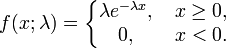While the cumulative distribution function can be found by: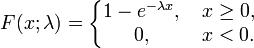exponential model: A function in the form of y = a·bx + c where a is non-zero and b is a positive value not equals to 1.

exradius: See eradius.

expression: A finite combination of symbols that are well-formed according to the rules applicable in the context at hand. Symbols can designate values (constants), variables, operations, relations, or can constitute punctuation or other syntactic entities.

exterior angle: The angle formed by one side, and the extension of an adjacent side of a polygon.

external angle: Also known as the exterior angle.

external force: A force on a system originating from an object outside the system under consideration. As opposed to internal force.

external tangent: The relation between two circles which intersect at exactly one point where the centres of neither circle is within the other circle. As opposed to internal tangent.

extraneous solution: An extra element of the solution set introduced during manipulation of equations.

extrapolation: Constructing new values by use of existing values whose arguments (the independent variable) are all greater or all less than (but not both) the new construction. As opposed to interpolation.

extrema: The maximum or minimum value of a function.

extreme value theorem: The theorem which states that a continuous function over an interval has and attains its extreme.

extremum: A non-descript maximum or minimum. The plural form of extremum is extrema.

A   B   C   D   E   F   G   H   I   J   K   L   M   N   O   P   Q   R   S   T   U   V   W   X   Y   Z